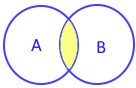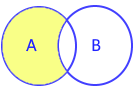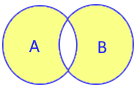# Set in Python

Collection of unordered and unindexed elements. No duplicate element is allowed.
Example : set of user IDs of any club membership, there can't be any duplicate values, they are not in any order and there is no indexing

## Declaring a set

``````my_set={'Alex','Ronald','John'}
print(my_set)``````
Output
``{'Alex', 'Ronald', 'John'}``

## Blank Set

``````my_set=set() # declaring a blank set
print(my_set)``````
We can't dsiplay elements based on position as set is unindexed.
``````my_set={"Alex","Ronald","John"}
print(my_set)``````
Above code will generate error
Python data structure using set an unordered unindexed and unique elements, methods and functions

## Displaying all items by looping

We used for loop to display all items of the set.
``````my_set={'Alex','Ronald','John'}
for i in my_set:
print(i)``````
Output is here
``````Alex
Ronald
John``````

## Searching element

``````my_set={'Alex','Ronald','John'}
if 'John' in my_set:
print("Yes, Present in set")
else:
print("No , not present in set")``````
Output is here
``Yes, Present in set``
Set Operations in Pythonunion() intersection() difference() symmetric_difference() A={1,2,3}B={3,4,5} A={1,2,3}B={3,4,5} A={1,2,3}B={3,4,5} A={1,2,3}B={3,4,5} A | B A.union(B) A & B A.intersection(B) A - B A.difference(B) A ^ BA.symmetric_difference(B) {1, 2, 3, 4, 5} {3} {1, 2} {1, 2, 4, 5}

Python set operations union, intersection, difference, symmetric_difference & updating the main set

## Add , remove , update elements

Methods of set object
 Method Description add(x) Add element x to the set update(x) Add iterable elements of x to the set clear() Removes all elements of the set copy() Creating a copy of the set discard() Remove element from the set isdisjoint() Check for presence of common element issubset() Check for presence of all elements issuperset() Check for presence of all elements pop() Remove random element from the set remove() Remove element from the set

## Number of elements in the set

`len()` returns number of elements present in a set
``````my_set={'Alex','Ronald','John'}
print("Number of elements: ",len(my_set))``````
Output is here
``Number of elements: 3``

## len(), max(),min(),sum()

``````my_set={5,3,7,9,4}
print("Maximum value : ",max(my_set))
print("Minimum value : ",min(my_set))
print("Number of elements : ",len(my_set))
print("Sum of elements : ",sum(my_set))``````
Output
``````Maximum value :  9
Minimum value :  3
Number of elements :  5
Sum of elements :  28``````

## Deleting set

``````my_set={'Alex','Ronald','John'}
del my_set
print("Number of elements: ",len(my_set))``````
The last line will create error as we are deleting the set before this line.

## Joint two sets by union()

We will use `union()` mehtod to join two sets and print final array
``````my_set1={'Alex','Ronald','John'}
my_set2={'Ron','Geek'}
my_set=my_set1.union(my_set2)
print(my_set)``````
Output is here
``{'Ronald', 'Geek', 'Alex', 'John', 'Ron'}``
We can use `difference()` to find out difference of first and second array with respect to first array.
``````my_set1={'Alex','Ronald','John'}
my_set2={'Ron','Ronald'}
my_set=my_set1.difference(my_set2)
print(my_set)``````
Output is here
``{'John', 'Alex'}``
We can use `intersection()` to get common element between two or more sets
``````my_set1={'Alex','Ronald','John'}
my_set2={'Ron','Alex'}
my_set=my_set1.intersection(my_set2)
print(my_set)``````
Output is
``{'Alex'}``
With three sets
``````my_set1={'a','b','c'}
my_set2={'d','b','a'}
my_set3={'f','b','a'}
my_set=my_set1.intersection(my_set2,my_set3)
print(my_set)``````
Output
``{'b', 'a'}``
`intersection_update()` only keeps common elements and removes other.
``````my_set1={'Alex','Ronald','John'}
my_set2={'Ron','Ronald'}
my_set1.intersection_update(my_set2)
print(my_set1)``````
Output is here
``{'Ronald'}``
`issubset()` returns true if all elements are present inside another set.
``````my_set1={'a','b','c'}
my_set2={'d','b','a','f','c'}
my_set=my_set1.issubset(my_set2)
print(my_set)``````
Output
``True``
`issuperset()` To get true if all elements of subset present in main set.
``````my_set1={'d','b','a','f','c'}
my_set2={'a','b','c'}
my_set=my_set1.issuperset(my_set2)
print(my_set)``````
Output
``True``

Subscribe to our YouTube Channel here

## Subscribe

* indicates required
Subscribe to plus2netplus2net.com